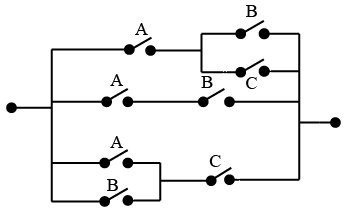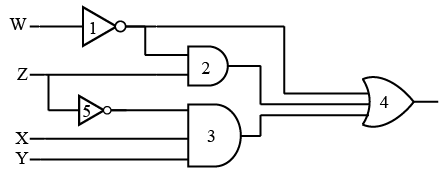# ISRO Scientist or Engineer Computer Science 2016

Instructions

For the following questions answer them individually

Question 1

# Which of the followingis true?Question 2

# What is the sum to infinity of the series,$$3+6x^{2}+9x^{4}+12x^{6}+$$........given $$\mid x$$ < 1 ?Question 3

# $$\lim_{x \rightarrow o} \frac{\sqrt{1+x}-\sqrt{1-x}}{x}$$ is given byQuestion 4

# If (G, -) is a group such that $$(ab)^{-1} =a_{-1} b^{-1}$$, $$\forall a,b \in G$$ then G is $$\frac{a}{an}$$Question 5

# A given connected graph G is a Euler Graphif and only if all vertices of G are ofQuestion 6

# Maximum number of edges in a n—node undirected graph withoutself-loops isQuestion 7

# The mimmum number of NAND gates required to implement the Boolean function $$A+A \bar B+A \bar B C$$ is equal toQuestion 8

# The minimum Boolean expression for the following circuit isQuestion 9

# For a binary half-subtractor having two inputs A and B, the correct set of logical expression for the outputs D (= A minus B) and X = borrow) areQuestion 10

# Consider the following gate networkWhich one of the following gates is redundant?OR